## 167. 两数之和 II - 输入有序数组

• 返回的下标值（index1 和 index2）不是从零开始的。
• 你可以假设每个输入只对应唯一的答案，而且你不可以重复使用相同的元素。

## 11. 盛最多水的容器522ms，13% 垫底了，别问，问就是暴力🤣

212ms，40%，利用双指针稍微优化了下，依然是遍历找每个柱的最大值，但是尾指针在移动时先判断下，如果比头指针大就直接 break，因为已经是最大值了，tail 是从右向左移动的## 42. 接雨水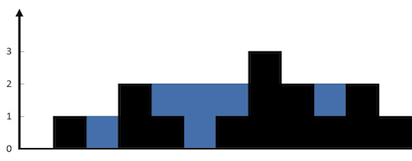2020/1/29 回顾

## 15. 三数之和## 283. 移动零

• 必须在原数组上操作，不能拷贝额外的数组。

• 尽量减少操作次数。

## 31. 下一个排列

• 第一步，逆序找到第一个峰值的左边第一个元素 `a[i-1]`

• 将峰值右边的最小的`a[i-1]`大的`a[j]`（其实就是`右边最后一个比它大的元素`) 元素与** a[i-1] **交换。

• 翻转刚刚调整过`a[i-1]`后面的逆序的数组 (`a[i]-->a[len-1]`)。## 41. 缺失的第一个正数

Update:2020.6.27

lc 上提交后的空间消耗居然比上面的还小一点😂

## 75. 颜色分类

• 一个直观的解决方案是使用计数排序的两趟扫描算法。
首先，迭代计算出 0、1 和 2 元素的个数，然后按照 0、1、2 的排序，重写当前数组。
• 你能想出一个仅使用常数空间的一趟扫描算法吗？

easy 题，对撞指针

## 345. 反转字符串中的元音字母

Write a function that takes a string as input and reverse only the vowels of a string.

Example 1:

Example 2:

Note:
The vowels does not include the letter “y”.

## 88. 合并两个有序数组

Given two sorted integer arrays nums1 and nums2, merge nums2 into nums1 as one sorted array.

Note:

• The number of elements initialized in nums1 and nums2 are m and n respectively.
• You may assume that nums1 has enough space (size that is greater or equal to m + n) to hold additional elements from nums2.

Example:

1ms ，98%beats.

（来自领扣）

## 118. 杨辉三角

Given a non-negative integer numRows, generate the first numRows of Pascal’s triangle.In Pascal’s triangle, each number is the sum of the two numbers directly above it.

Example:

## 119. 杨辉三角 II

Given a non-negative index k where k ≤ 33, return the kth index row of the Pascal’s triangle.

Note that the row index starts from 0.In Pascal’s triangle, each number is the sum of the two numbers directly above it.

Example:

Could you optimize your algorithm to use only O(k) extra space?

## 59. 螺旋矩阵 II

UPDATE(2020.12.17)：更新了通用的解法，对应 AcWing756. 蛇形矩阵，美化了下代码

Example 1:

Example 2:

## 498. 对角线遍历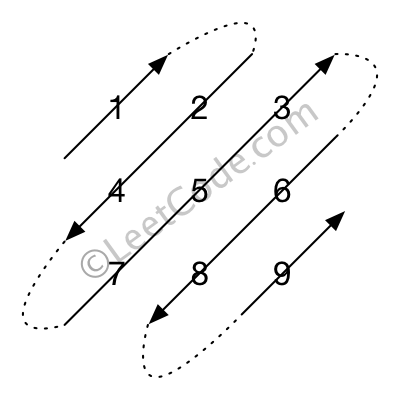1. 给定矩阵中的元素总数不会超过 100000 。

## 1424. 对角线遍历 II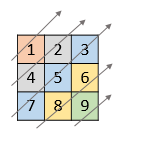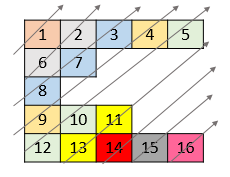• `1 <= nums.length <= 10^5`
• `1 <= nums[i].length <= 10^5`
• `1 <= nums[i][j] <= 10^9`
• `nums` 中最多有 `10^5` 个数字。

186th 周赛的 t3，还是挺有意思的，这题我拿到的第一想法其实是找一下关系直接排序，但是实际上有更好的方法

## 215. 数组中的第 K 个最大元素

Find the kth largest element in an unsorted array. Note that it is the kth largest element in the sorted order, not the kth distinct element.

Example 1:

Example 2:

Note:
You may assume k is always valid, 1 ≤ k ≤ array’s length.

70%左右的 beat，当时感觉还行，时间复杂度应该是`O(KlogN)`，后来越想越不对，又去看了下堆排序，发现我之前写的堆排序都是有问题的

95% beat，比上面的要快很多，相比之前的方法，构造堆的方式发生了变化，上面那种通过自上而下的 insert 方式时间复杂度是 O(NlogN)，其实想想，这两种方式是完全相反的，insert 的方式，最后一层每个元素最坏都可能调整`logN`次，而最后一层也是元素最多的一层，这样一来复杂度就会大大增加，相反如果采用从底向上的`swim`方式最后一层都只需要调整`1`次，而根节点需要调整`logN`次，而根节点只有一个，时间复杂度就会大大降低，最终的时间复杂度就是`O(N)`具体推算可以看这篇文章， 现在的时间复杂度才真的是`O(KlogN)`💥💥 上面这两种做法是有问题的，失去了用堆的优势，大根堆的做法必须要阿将整个堆构建完成后才能去找 topk 这样的话内存消耗比较大，应该维护一个小根堆，这样如果数据量很大的时候不用全读入内存中， 这题因为是我自己实现的堆，所以建堆的复杂度是 O(N)（如果使用官方的 API，建堆的时间复杂度就是 NlogN），最终大根堆小根堆复杂度取决于 K 和 N 的大小关系，但是面试的时候最好不要说用大根堆的做法

2ms，99%beat，一般情况下的 topK 问题，如果用堆解决的话应该都是采用小根堆这种做法来做，时间复杂度为`O(NlogK)`，维护一个大小为 k 的小根堆，然后再遍历后面 n-k 个元素，依次和当前最小堆的堆顶比较（当前 topK 中的最小元素，堆顶），如果比它小就和它交换然后调整堆，这样就始终保持了这个堆是当前的 topK 小，最后的堆顶就是第 K 大的元素。

ACWing 上交的，wa 了好几次，发现是二分写错了，哎，二分真难，其实还可以做一下随机处理

Update: 2020.6.28

BFPRT 算法 大佬们提出来的根据上面快排改进而来，其实面试把小根堆和快排的解法答出来应该就差不多了，这个解法还是有些不容易写出来## 347. 前 K 个高频元素

`nums=[1,1,1,2,2,2,3,3,3] ，k=2` 这样的就是有歧义的

## 295. 数据流的中位数

[2,3,4] 的中位数是 3

[2,3] 的中位数是 (2 + 3) / 2 = 2.5

• void addNum(int num) - 从数据流中添加一个整数到数据结构中。
• double findMedian() - 返回目前所有元素的中位数。

• 如果数据流中所有整数都在 0 到 100 范围内，你将如何优化你的算法？
• 如果数据流中 99% 的整数都在 0 到 100 范围内，你将如何优化你的算法？

## 415. 字符串相加

1. num1 和 num2 的长度都小于 5100.
2. num1 和 num2 都只包含数字 0-9.
3. num1 和 num2 都不包含任何前导零。
4. 你不能使用任何內建 BigInteger 库， 也不能直接将输入的字符串转换为整数形式。

## 43. 字符串相乘

1. num1 和 num2 的长度小于 110。
2. num1 和 num2 只包含数字 0-9。
3. num1 和 num2 均不以零开头，除非是数字 0 本身。
4. 不能使用任何标准库的大数类型（比如 BigInteger）或直接将输入转换为整数来处理。## 8. 字符串转换整数 (atoi)

1. 这样正数的边界就是`-INT_MAX`, 负数是`INT_MIN`

2. 然后我们同样也用负数来保存结果，`res=res\*10-digit`

3. 我们需要保证这个值是在`INT`范围内的，也就是 res*10-digit>=limit （负边界）

4. 所以我们需要对`res`做判断，但是直接判断可能会溢出，所以进行移项，变换为 `res<(limit+digit)/10`

5. 最后如果是正数就返回 `-res`, 负数就返回`res`

## 1071. 字符串的最大公因子

`k' < k`，而根据题意`k'`也为`l1, l2` 的公约数，则`k'`必能被`k`整除，这说明我们可以将该字串的长度扩充到`k`，同时保持它仍然为`str1``str2`的公因子，所以这种情况下这个长度为`k`的公因子就不是最大公因子。
`k' > k`， 根据题意`k'``l1`, `l2` 的公约数， 而 k 为最大公约数，而这时出现了一个比最大公约数还大的公约数，这是矛盾的，所以这种情况也是不可能的。

## 914. 卡牌分组

• 每组都有 X 张牌。
• 组内所有的牌上都写着相同的整数。

• `1 <= deck.length <= 10000`
• `0 <= deck[i] < 10000`

wa 一次就知道咋做了，一开始以为只要所有元素出现次数可以整除就行了，wa 了之后就意识到只要求一个最大公约数就可以了

## 165. 比较版本号

. 字符不代表小数点，而是用于分隔数字序列。

1. 版本字符串由以点 （.） 分隔的数字字符串组成。这个数字字符串可能有前导零。
2. 版本字符串不以点开始或结束，并且其中不会有两个连续的点。

## 189. 旋转数组

• 尽可能想出更多的解决方案，至少有三种不同的方法可以解决这个问题。
• 要求使用空间复杂度为 O(1) 的 原地 算法

`O(N)` 应该是最优解了

## 1232. 缀点成线

10.20 竞赛第一题，判断给定的点是不是再一条直线上，判断和前两个点是不是在一条直线上，注意不要直接除算斜率，那样是不准确的

## 1233. 删除子文件夹

• 如果文件夹 `folder[i]` 位于另一个文件夹 `folder[j]` 下，那么 `folder[i]` 就是 `folder[j]` 的子文件夹。
文件夹的「路径」是由一个或多个按以下格式串联形成的字符串：

• `/` 后跟一个或者多个小写英文字母。
例如，`/leetcode``/leetcode/problems` 都是有效的路径，而空字符串和 `/` 不是。

• 1 <= folder.length <= 4 * 10^4
• 2 <= folder[i].length <= 100
• folder[i] 只包含小写字母和 /
• folder[i] 总是以字符 / 起始
• 每个文件夹名都是唯一的

`2019.10.20`的竞赛题，当时没做出来。一直超时，太菜了

`folder = ["/a","/a/b","/c/d","/c/d/e","/c/f"]` 题目其实也在暗示我们要排序，给的 case 都是排好序的

Difficulty: 困难

## 680. 验证回文字符串 Ⅱ

1. 字符串只包含从 a-z 的小写字母。字符串的最大长度是 50000。

## 1332. 删除回文子序列

「子序列」定义：如果一个字符串可以通过删除原字符串某些字符而不改变原字符顺序得到，那么这个字符串就是原字符串的一个子序列。

「回文」定义：如果一个字符串向后和向前读是一致的，那么这个字符串就是一个回文。

• `0 <= s.length <= 1000`
• `s` 仅包含字母 ‘a’ 和 ‘b’

## 435. 无重叠区间

• 可以认为区间的终点总是大于它的起点。
• 区间 [1,2] 和 [2,3] 的边界相互“接触”，但没有相互重叠。

171ms，8%，感觉快要过不了了。本来是是写的记忆化递归的，结果过不了。卡在倒数第二个 case 上

## 263. 丑数

1. `1` 是丑数。
2. 输入不会超过 `32` 位有符号整数的范围：`[−231, 231 − 1]`

## 1333. 餐厅过滤器

• `1 <= restaurants.length <= 10^4`
• `restaurants[i].length == 5`
• `1 <= idi, ratingi, pricei, distancei <= 10^5`
• `1 <= maxPrice, maxDistance <= 10^5`
• `veganFriendlyi``veganFriendly` 的值为 0 或 1 。
• 所有 `idi` 各不相同。

177 周赛的 T1

copy 大佬的原生解法

## 151. 翻转字符串里的单词

• 无空格字符构成一个单词。
• 输入字符串可以在前面或者后面包含多余的空格，但是反转后的字符不能包括。
• 如果两个单词间有多余的空格，将反转后单词间的空格减少到只含一个。

1. 翻转整个字符
2. 翻转单个字符
3. 去除多余空格

case 就不粘了

O(1) 进阶版有时间再来补

## 5341. 最后 K 个数的乘积

• 将数字 num 添加到当前数字列表的最后面。
1. getProduct(int k)
• 返回当前数字列表中，最后 k 个数字的乘积。
• 你可以假设当前列表中始终 至少 包含 k 个数字。
• 题目数据保证：任何时候，任一连续数字序列的乘积都在 32-bit 整数范围内，不会溢出。

176 周赛的第二题，思路倒是很容易想到，维护一个前缀积，然后用全积除以对应 k 位置的就行了，但是细节的处理出了大问题 haha~

## 面试题 64. 求 1+2+…+n

`1+2+...+n` ，要求不能使用乘除法、for、while、if、else、switch、case 等关键字及条件判断语句（A?B:C）。

• 1 <= n <= 10000

## 470. 用 Rand7() 实现 Rand10()## 1103. 分糖果 II

• `1 <= candies <= 10^9`
• `1 <= num_people <= 1000`

## 1013. 将数组分成和相等的三个部分

1. 3 <= A.length <= 50000
2. -10^4 <= A[i] <= 10^4

## 836. 矩形重叠

1. 两个矩形 `rec1``rec2` 都以含有四个整数的列表的形式给出。
2. 矩形中的所有坐标都处于 `-10^9``10^9` 之间。
3. `x` 轴默认指向右，`y` 轴默认指向上。
4. 你可以仅考虑矩形是正放的情况。

## 892. 三维形体的表面积

• `1 <= N <= 50`
• `0 <= grid[i][j] <= 50`

## 289. 生命游戏

1. 如果活细胞周围八个位置的活细胞数少于两个，则该位置活细胞死亡；

2. 如果活细胞周围八个位置有两个或三个活细胞，则该位置活细胞仍然存活；

3. 如果活细胞周围八个位置有超过三个活细胞，则该位置活细胞死亡；

4. 如果死细胞周围正好有三个活细胞，则该位置死细胞复活；

• 你可以使用原地算法解决本题吗？请注意，面板上所有格子需要同时被更新：你不能先更新某些格子，然后使用它们的更新后的值再更新其他格子。
• 本题中，我们使用二维数组来表示面板。原则上，面板是无限的，但当活细胞侵占了面板边界时会造成问题。你将如何解决这些问题？

bugfree

## 171. Excel 表列序号

``````A -> 1
B -> 2
C -> 3
...
Z -> 26
AA -> 27
AB -> 28
...
``````

go 写法

## 724. 寻找数组的中心索引

• `nums` 的长度范围为 `[0, 10000]`
• 任何一个 `nums[i]` 将会是一个范围在 `[-1000, 1000]`的整数。

## 945. 使数组唯一的最小增量

1. `0 <= A.length <= 40000`
2. `0 <= A[i] < 40000`

## 面试题 45. 把数组排成最小的数

• `0 < nums.length <= 100`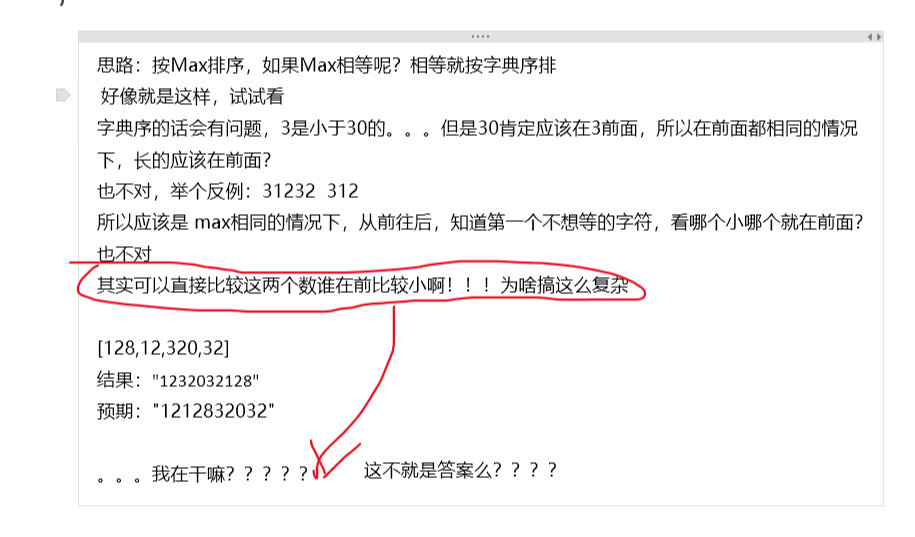## 179. 最大数

`func Slice(slice interface{}, less func(i, j int) bool)`

Difficulty: 中等

## 172. 阶乘后的零

Difficulty: 简单## 793. 阶乘函数后 K 个零

Difficulty: 困难

`f(x)` 是 `x!` 末尾是 0 的数量。（回想一下 `x! = 1 * 2 * 3 * ... * x`，且`0! = 1`

• `K`是范围在 `[0, 10^9]` 的整数

## 73. 矩阵置零

Difficulty: 中等

• 一个直接的解决方案是使用  O(mn) 的额外空间，但这并不是一个好的解决方案。
• 一个简单的改进方案是使用 O(m+n) 的额外空间，但这仍然不是最好的解决方案。
• 你能想出一个常数空间的解决方案吗？

## 面试题 16.11. 跳水板

Difficulty: 简单

• 0 < shorter <= longer
• 0 <= k <= 100000

tag 里面有递归，记忆化什么的。加上看见群友的讨论，又先入为主了，唉，写了半天的回溯，想着怎么去重，突然意识到直接一个循环就能解决了。菜啊

## NC82. 苹果树

• 1 <= a[i] , b[i] <= 1e9
• 1 <= len(a), len(b) <= 1e5

## 453. 最小移动次数使数组元素相等

Difficulty: 简单

n-1 个元素+1，就相当于 1 个元素-1，思维的转换，题目就变得简单了

## 696. 计数二进制子串

Difficulty: 简单

• `s.length` 在 1 到 50,000 之间。
• `s` 只包含“0”或“1”字符。

## 844. 比较含退格的字符串

Difficulty: 简单

1. `1 <= S.length <= 200`
2. `1 <= T.length <= 200`
3. `S``T` 只含有小写字母以及字符 `'#'`

• 你可以用 `O(N)` 的时间复杂度和 `O(1)` 的空间复杂度解决该问题吗？

O（N）空间的就不写了，随便搞搞就行了，关键是 O(1) 空间的解法，这里核心就是双指针从后想前扫描，然后注意边界就 ok 了